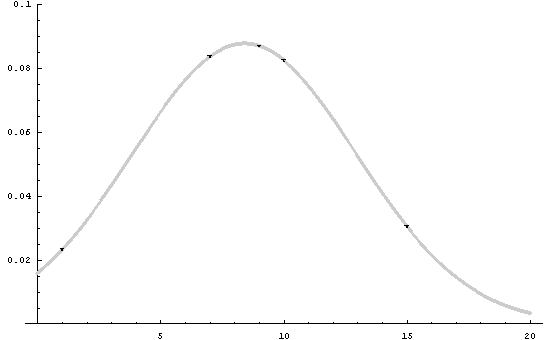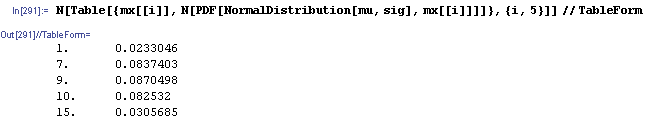# Hex, Bugs and More Physics | Emre S. Tasci

a blog about physics, computation, computational physics and materials…

## A (Lame) Proof of the Probability Sum Rule

### December 21, 2007 Posted by Emre S. Tasci

Q: Prove the Probability Sum Rule, that is: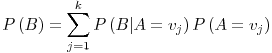(where A is a random variable with arity (~dimension) k) using Axioms:and assuming: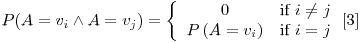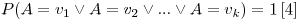We will first prove the following equalities to make use of them later in the actual proof: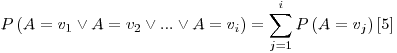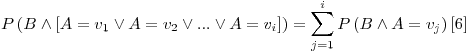Proof of :

Using , we can write: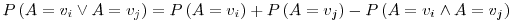where, from the assumption , we have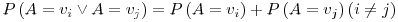Using this fact, we can now expand the LHS of  as: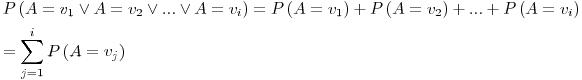Proof of :

Using the distribution property, we can write the LHS of  as: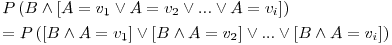from , it is obvious that: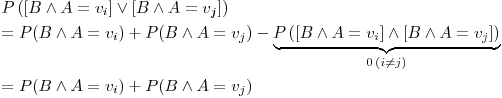then using , we can rearrange and rewrite the LHS of  as: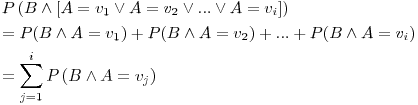Final Proof :

Before proceeding, I will include two assumptions about the Universal Set (this part is the main reason for the proof to be lame by the way).

Define the universal set U as the set that includes all possible values of some random variable X. All the other sets are subsets of U and for an arbitrary set A, we assume that:Furthermore, we will be assuming that, a condition that includes all the possible outcomes(/values) for a variable A is equivalent to the universal set. Let A have arity k, then:You can think of the condition (U) as the definite TRUE 1.

Now we can begin the proof of the equalityusing the Bayes Rule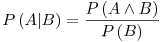we can convert the inference relation into intersection relation and rewrite RHS as: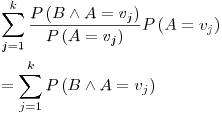using , this is nothing but:## Boasting? I guess so… 8)

### Posted by Emre S. Tasci

Suppose that you’ve collected some data from the output of a program. Let’s say that some part of this data consists of Author names something similar to: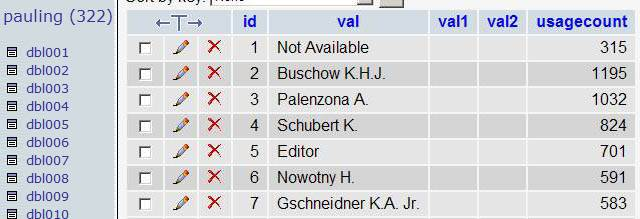You want to split the initials from the surnames. This is piece of cake with PHP but I don’t want to go parsing each row of which there are many… So, take a look at this ugly beauty:

UPDATE dbl004 set val1 = IF(LOCATE(".",val),TRIM(SUBSTRING(SUBSTRING_INDEX(val,".",1),1, LENGTH(SUBSTRING_INDEX(val,".",1)) – LENGTH(SUBSTRING_INDEX(SUBSTRING_INDEX(val,".",1)," ",-1)))),val), val2 = IF(LOCATE(".",val), TRIM(SUBSTRING(val,LENGTH(SUBSTRING_INDEX(val,".",1)) – LENGTH(SUBSTRING_INDEX(SUBSTRING_INDEX(val,".",1)," ",-1)))),"");

aaaaand here is what you get: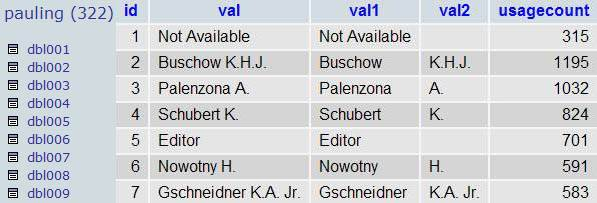if you are thinking something similar to

UPDATE dbl004 SET val1 = LEFT(val,LOCATE(" ",val)-1), val2 = RIGHT(val,LENGTH(val)-LOCATE(" ",val));

or

UPDATE dbl004 set val1 = TRIM(SUBSTRING(SUBSTRING_INDEX(val,".",1),1,LENGTH(SUBSTRING_INDEX(val,".",1)) – LENGTH(SUBSTRING_INDEX(SUBSTRING_INDEX(val,".",1)," ",-1)))), val2 = TRIM(SUBSTRING(val, LENGTH(SUBSTRING_INDEX(val,".",1)) – LENGTH(SUBSTRING_INDEX(SUBSTRING_INDEX(val,".",1)," ",-1))));

Try to process these 3 values: "van der Graaf K.L. Jr.", "Not Available" and "Editor".

About this entry: I couldn’t refrain myself from boasting after I managed to come up with that beautiful MySQL query… sorry for that. (Yes, I know, superbia, the 7th and the most deadly…) So let me try to balance this arrogant entry of mine:

With my best regards,

## SAGE: Open Source Mathematics Software

### December 9, 2007 Posted by Emre S. Tasci

I don’t know how it is but it offers pretty much and does this in the free spirit so I’m oughta give this software a try. Will inform you when I cover some bases…## Some “trivial” derivations

### December 4, 2007 Posted by Emre S. Tasci

Information Theory, Inference, and Learning Algorithms by David MacKay, Exercise 22.5:

A random variable x is assumed to have a probability distribution that is a mixture of two Gaussians,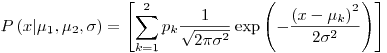where the two Gaussians are given the labels k = 1 and k = 2; the prior probability of the class label k is {p1 = 1/2, p2 = 1/2};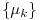are the means of the two Gaussians; and both have standard deviation sigma. For brevity, we denote these parameters byA data set consists of N points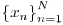which are assumed to be independent samples from the distribution. Let kn denote the unknown class label of the nth point.

Assuming thatand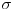are known, show that the posterior probability of the class label kn of the nth point can be written asand give expressions for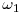and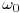.

Derivation: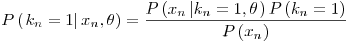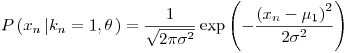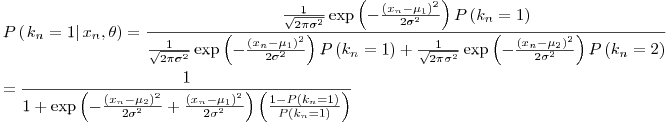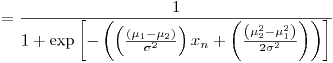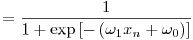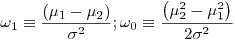Assume now that the meansare not known, and that we wish to infer them from the data. (The standard deviationis known.) In the remainder of this question we will derive an iterative algorithm for finding values forthat maximize the likelihood,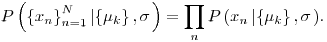Let L denote the natural log of the likelihood. Show that the derivative of the log likelihood with respect to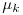is given by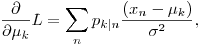where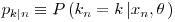appeared above.

Derivation: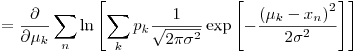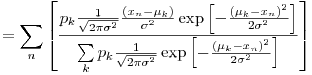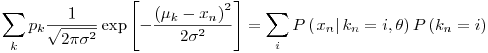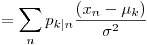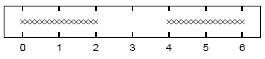Assuming that=1, sketch a contour plot of the likelihood function as a function of mu1 and mu2 for the data set shown above. The data set consists of 32 points. Describe the peaks in your sketch and indicate their widths.

Solution:

We will be trying to plot the functionif we designate the function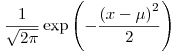as p[x,mu] (remember that=1 and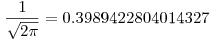),then we have:And in Mathematica, these mean:

mx=Join[N[Range[0,2,2/15]],N[Range[4,6,2/15]]]
Length[mx]
ListPlot[Table[{mx[[i]],1},{i,1,32}]]p[x_,mu_]:=0.3989422804014327 * Exp[-(mu-x)^2/2];
pp[x_,mu1_,mu2_]:=.5 (p[x,mu1]+p[x,mu2]);
ppp[xx_,mu1_,mu2_]:=Module[
{ptot=1},
For[i=1,i<=Length[xx],i++,
ppar = pp[xx[[i]],mu1,mu2];
ptot *= ppar;
(*Print[xx[[i]],"\t",ppar];*)
];
Return[ptot];
];

Plot3D[ppp[mx,mu1,mu2],{mu1,0,6},{mu2,0,6},PlotRange->{0,10^-25}];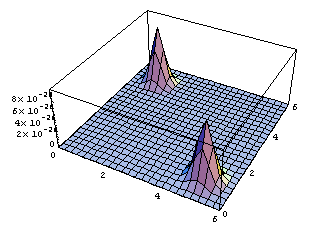ContourPlot[ppp[mx,mu1,mu2],{mu1,0,6},{mu2,0,6},{PlotRange->{0,10^-25},ContourLines->False,PlotPoints->250}];(*It may take a while with PlotPoints->250, so just begin with PlotPoints->25 *)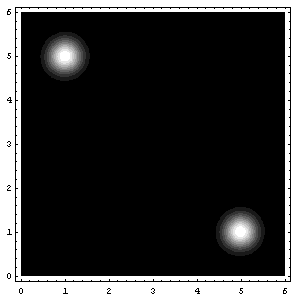That’s all folks! (for today I guess 8) (and also, I know that I said next entry would be about the soft K-means two entries ago, but believe me, we’re coming to that, eventually 😉

Attachments: Mathematica notebook for this entry, MSWord Document (actually this one is intended for me, because in the future I may need them again)

## Likelihood of Gaussian(s) – Scrap Notes

### December 3, 2007 Posted by Emre S. Tasci

Given a set of N data x,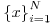,  the optimal parameters for a Gaussian Probability Distribution Function defined as: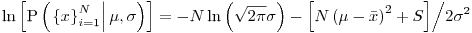are: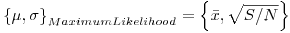with the definitionsLet’s see this in an example:

Define the data set mx:
mx={1,7,9,10,15}

Calculate the optimal mu and sigma:
dN=Length[mx];
mu=Sum[mx[[i]]/dN,{i,1,dN}];
sig =Sqrt[Sum[(mx[[i]]-mu)^2,{i,1,dN}]/dN];
Print["mu = ",N[mu]];
Print["sigma = ",N[sig]];

Now, let’s see this Gaussian Distribution Function:
<<StatisticsNormalDistribution
ndist=NormalDistribution[mu,sig];

<<GraphicsMultipleListPlot`
MultipleListPlot[Table[{x,PDF[NormalDistribution[mu,sig],x]}, {x,0,20,.04}],Table[{mx[[i]], PDF[NormalDistribution[mu,sig],mx[[i]]]},{i,5}], {PlotRange->{Automatic,{0,.1}},PlotJoined->{False,False}, SymbolStyle->{GrayLevel[.8],GrayLevel}}]